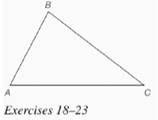Chapter 7.CR, Problem 22CRElementary Geometry For College St...

7th Edition
Alexander + 2 others
ISBN: 9781337614085

Solutions

Chapter
SectionElementary Geometry For College St...

7th Edition
Alexander + 2 others
ISBN: 9781337614085
Textbook Problem

In Review Exercises 18 to 23, use construction methods with the accompanying figure.Use the result from Review Exercise 18 to inscribe a circle in Δ A B C .To determine

To inscribe: A circle in triangle ABC with the incenter.

Explanation

Given:

The triangle ABC is shown below.

.

Calculation:

Consider the triangle ABC.

The incenter I of the triangle ABC is shown below

Having found the incenter I, we need the length of the radius to draw a circle.

Since IN¯ is perpendicular to

Still sussing out bartleby?

Check out a sample textbook solution.

See a sample solution

The Solution to Your Study Problems

Bartleby provides explanations to thousands of textbook problems written by our experts, many with advanced degrees!

Get Started

In Exercises 2730, determine all values of x for which each function is discontinuous. 30. f(x) = |2x|x

Applied Calculus for the Managerial, Life, and Social Sciences: A Brief Approach

Evaluate the integrals in Problems 7-36. Check your results by differentiation. 20.

Mathematical Applications for the Management, Life, and Social Sciences

Evaluate each expression: 16+4(7+8)3

Elementary Technical Mathematics

Find the area of the region bounded by the given curves. y = x2, y = 4x x2

Single Variable Calculus: Early Transcendentals, Volume I

Find the domain of the function. 32. f(x)=2x35x2+x6

Single Variable Calculus: Early Transcendentals

f(x) = x3 2x2 + x 1 has a local maximum at: a) 0 b) 1 c) 13 d) f has no local maxima

Study Guide for Stewart's Single Variable Calculus: Early Transcendentals, 8th